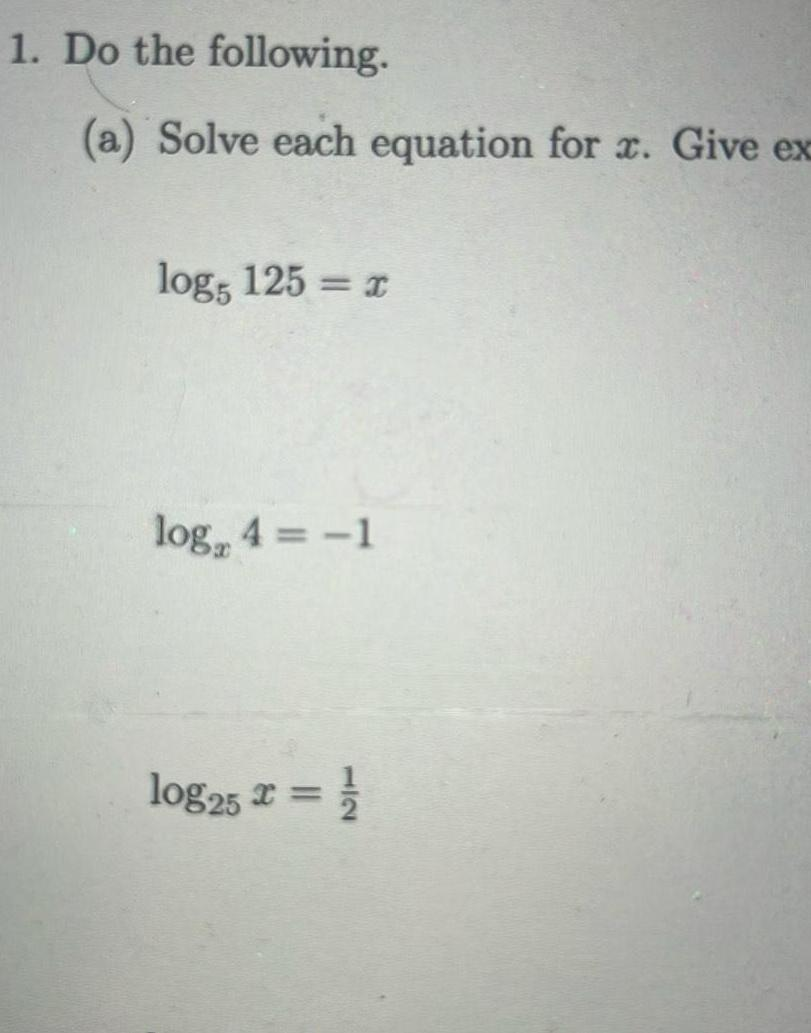Question:

# 1 Do the following a Solve each equation for x Give ex log5

Last updated: 11/20/20231 Do the following a Solve each equation for x Give ex log5 125 x log 4 1 log25 x